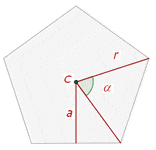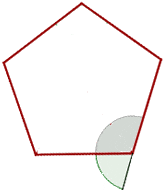Chapters

## Introduction

A polygon refers to a two-dimensional geometrical figure that contains a finite number of sides. The straight line segments that join each other from one end to another make up the sides of a polygon. These line segments are known as edges or sides. The angles of the polygons are formed at the points where the ends of two line segments join each other. These points are referred to as vertex or corners of the polygons. A simple example of a polygon can be a triangle that has three sides. Although a circle is a two-dimensional figure, however, we cannot say that it is a polygon because of its curved shape and absence of sides or angles. From this, we can conclude that all the polygons are two-dimensional shapes, but all two-dimensional shapes are not polygons.The best Maths tutors available4.9 (36 reviews)
Intasar
£48
/h1st lesson free!4.9 (29 reviews)
Paolo
£30
/h1st lesson free!5 (16 reviews)
Jamie
£25
/h1st lesson free!5 (16 reviews)
Harinder
£15
/h1st lesson free!5 (32 reviews)
Sehaj
£25
/h1st lesson free!5 (17 reviews)
Matthew
£30
/h1st lesson free!4.9 (8 reviews)
Gian lorenzo
£65
/h1st lesson free!4.9 (12 reviews)
Petar
£40
/h1st lesson free!4.9 (36 reviews)
Intasar
£48
/h1st lesson free!4.9 (29 reviews)
Paolo
£30
/h1st lesson free!5 (16 reviews)
Jamie
£25
/h1st lesson free!5 (16 reviews)
Harinder
£15
/h1st lesson free!5 (32 reviews)
Sehaj
£25
/h1st lesson free!5 (17 reviews)
Matthew
£30
/h1st lesson free!4.9 (8 reviews)
Gian lorenzo
£65
/h1st lesson free!4.9 (12 reviews)
Petar
£40
/h1st lesson free!

## What is a Polygon?

A polygon is defined as:

A closed figure that is composed of several line segments instead of the curves in the two-dimensional plane is known as a polygon

The word polygon is made up of two words: Poly and gon. Poly means many and gon means sides. So, a polygon is a figure that has multiple sides. We know that we need at least three line segments to make a closed figure. Hence, a polygon with three sides and three angles is known as a triangle. Some other examples of polygons include pentagon, hexagon, quadrilateral, and octagon.

## Properties of Polygon

Depending on the sides and angles, all polygons have the following properties:

• The aggregate of all the interior angles of an n-sided polygon is equal to• The number of diagonals in an n-sided polygon is given by• The measure of an interior angle of a regular polygon with n sides is given by• To calculate the number of triangles that are formed when the diagonals  are joined from one corner of a polygon, we use the formula n - 2
• The measure of the exterior angle of a regular polygon with n sides is given by## Types of Polygon

Polygons are divided into several different categories based on their sides and angles. These categories are:

• Regular polygon
• Irregular polygon
• Concave polygon
• Convex polygon

In the next section of the article, we will focus on a regular polygon.

## Regular Polygon

A regular polygon is defined as:

A polygon in which all sides and interior angles are equal is known as a regular polygon

Some examples of regular polygons are:

• Equilateral triangle
• Rhombus
• Square

If all the sides and interior angles of the polygon are equal, then it is known as a regular polygon. Examples of regular polygons include square, rhombus, equilateral triangle, etc. One such regular polygon is shown below that has six sides and six interior angles.Regular polygons can be inscribed in circles.

## Elements of a Regular Polygon

In this section, we will discuss the important elements of a regular polygon. Consider the following regular polygon:Its main elements are center, radius and apothem. Now, we will see one by one what are the properties of these elements.

### Center

The center is the inner point equidistant from each vertex. It means that a center "c" as shown in the above figure is located at an equal distance from each of the five vertices.

The radius, r, is the segment that goes from the center to each vertex.

### Apothem

The apothem, a, is the distance from the center to the midpoint of one side.

## Central Angle of a Regular Polygon

The central angle of a regular polygon is formed by two consecutive radii as shown in the figure below:If the polygon has n number of sides, then the central angle can be calculated by dividingby n. For instance, a pentagon has 5 sides, so its central angle will be. Similarly, a hexagon has six sides, so its central angle will be## Interior Angle of a Regular Polygon

The interior angle of a regular polygon is formed by two consecutive sides as shown in the figure below:To calculate the interior angle of a regular polygon, you should know the central angle. The formula for computing the interior angle of a regular polygon is given below:
Interior angle =- central angle
We have already calculated the central angle of a regular pentagon which is also a regular polygon. From its central angle, we can easily calculate the interior angle of the polygon like this:
Interior angle of pentagon =- central angle
=Similarly, the central angle of the hexagon was, so its interior angle will be:
Interior angle of the hexagon =## Exterior Angle of a Regular Polygon

The exterior angle of a regular polygon is formed by a side and the extension of a consecutive side as shown in the figure below:The exterior and interior angles are supplementary which means that they add up to 180º. This shows that the exterior angles are equal to the central angles. So, we can say that the general rule of the thumb is:
Exterior angle of the regular polygon = central angle of the regular polygon
The central angle of a regular pentagon is, so the exterior angle is also. Similarly, the central angle of the regular hexagon is, so its exterior angle is also.

## Perimeter of a Regular Polygon

The perimeter is equal to the sum of the lengths of all sides or the length of a side multiplied by the number of sides.

## Area of a Regular Polygon

The area of a regular polygon is equal to half of the product of perimeter and apothem. The formula for an area of the regular polygon is given below:

Area =## Example

Calculate the perimeter and area of the hexagon:First, we will calculate the perimeter of the above hexagon which is also a regular polygon because it has the same number of sides and angles. We know that the perimeter of the regular polygon is equal to the sum of the length of its all sides. One side of the above polygon is 12 cm and the number of sides is 6. So, the perimeter is equal to :
Perimeter = 6 x 12 = 72 cm
Now, we will calculate the area of the above polygon. The area of a regular polygon is equal to half of the product of perimeter and apothem. The perimeter of the above polygon is 72cm and apothem is given in the figure which is 8.4 cm. Hence, we will substitute these values of perimeter and apothem in the below formula to get the area:
Area ==The platform that connects tutors and students4.00 (2 rating(s))Loading...